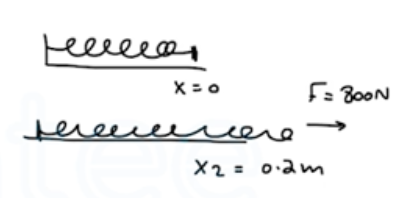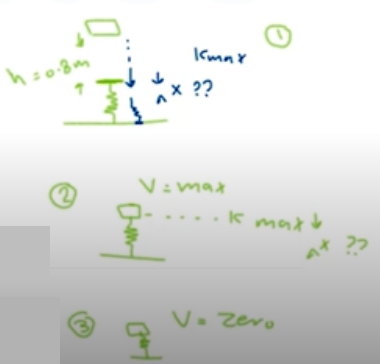Need Help?

Subscribe to Physics 1

###### \${selected_topic_name}
• Notes

$\begin{array}{l}{\text { A force of } 800 \mathrm{N} \text { stretches a certain spring a distance of }} \\ {0.200 \mathrm{m} . \text { (a) What is the potential energy of the spring when it is }} \\ {\text { stretched } 0.200 \mathrm{m} ? \text { (b) What is its potential energy when it is com- }} \\ {\text { pressed } 5.00 \mathrm{cm} ?}\end{array}$$U_{e{1}}=\frac{1}{2} k {x_{1}}^{2}=0$

$U_{1}=0$

$U_{2}=\frac{1}{2} k{ x_{2}}^{2}=\frac{1}{2} k(0.2)^{2}$

$F=k x \rightarrow k=\frac{F}{x}$

$U_{2}=\frac{1}{2}\left(\frac{F}{x}\right) x^{2} \rightarrow \frac{1}{2} F x=\frac{1}{2}(800)(0.2)=80J$$U_{3}=\frac{1}{2} k {x_{3}}^{2}$

${k=\frac{\mathrm{F}}{\mathrm{x}}=\frac{800}{0.2}=4000 \mathrm{N} / \mathrm{m}}$

$U_{3}=\frac{1}{2}(4000)(-0.05)^2=5 J$

$U_{2}=\frac{1}{2} k {x_{2}}^{2} \quad, \quad U_{3}=\frac{1}{2} k{ x_{3}}^{2}$

$\frac{U_{2}}{U_{3}}=\frac{\frac{1}{2} {x_{2}}^{2}}{\frac{1}{2}k{ x_{3}}^{2}}=\frac{{x_{2}}^{2}}{{x_{3}}^2}$

$U_{3}=U_{2}\left(\frac{{x_{3}}^{2}}{{x_{2}}^{2}}\right)=80\left(\frac{-0.05^{2}}{0.22}\right)$

$∴ U_{3}=5 \mathrm{J}$

$\begin{array}{l}{\text { A spring of negligible mass has force constant } k=} \\ {1600 \mathrm{N} / \mathrm{m} . \text { ( a) How far must the spring be compressed for } 3.20 \mathrm{J}} \\ {\text { of potential energy to be stored in it? (b) You place the spring ver- }} \\ {\text { tically with one end on the floor. You then drop a } 1.20-\mathrm{kg} \text { book }} \\ {\text { onto it from a height of } 0.80 \mathrm{m} \text { above the top of the spring. Find }} \\ {\text { the maximum distance the spring will be compressed. }}\end{array}$

$U=\frac{1}{2} k x^{2} \longrightarrow x=\sqrt{\frac{2 U}{k}}=\sqrt{\frac{2(3.2)}{1600}}=0.0632 \mathrm{m}$

$∴ x=6.32 \mathrm{cm}$$K_1+U_{1}+W_{other}=K_f+U_f$

$U_{1}=m g(h+{x})$

$U_{2}=U_{e}=\frac{1}{2} K x^{2}$

$U_{1}=(1.2)(9.8)(0.8+x)$

$U_{2}=\frac{1}{2}(1600) x^{2}$

$U_{1}=U_{f} \rightarrow (1.2)(9.8)(0.8+x)=\frac{1}{2}(1600)x^2$

$\rightarrow 9.408+11.76 x=800 x^{2}$

$\rightarrow 800 x^ 2-11.76 x-9.408=0 \rightarrow x=0.12 m$

$=12 \mathrm{cm}$